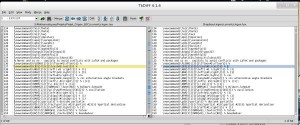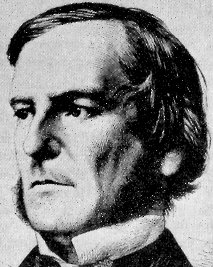# Month: January 2012Diff is a standard utility from the Unix world outputting the differences between two files. Even if diff can handle binary files, it is used mostly to compare two versions of a text file. For mathematicians, diff is helpful for the comparison of $\LaTeX$ files written with co-authors, in particular via its graphical user interface tkdiff written in Tcl/Tk. If you use GNOME, you make take a look at meld. There is also diffpdf, which is very convenient to compare two versions of a PDF file. There is another well known utility called patch, a sort of reversed diff, used millions of times a day for software development, worldwide. On the technical side, diff relies on an algorithm for solving the longest common subsequence problem. By the way, if you are curious about text algorithms, you may take a look at the (free!) books written by Maxime Crochemore, from Marne-la-Vallée.

Last Updated on 2014-06-17Have you heard about the Go and the Rust system programming languages? Both are statically typed with a syntax not so far from the one of C/C++. They come with garbage collector, generic programming, and modules. They implement polymorphism without classes and support Unicode (UTF-8) by default. Go was created in 2007 and is supported by Google, while Rust was created in 2006 and is supported by the Mozilla Labs. The programming languages C/C++ are Industry standards. Writing in C/C++ provides high portability and access to a wide range of libraries and optimized compilers.  However, it is quite easy to write buggy and/or unmaintainable code in C/C++. The aim of recent programming languages such as Go and Rush is to make system programming easier and smarter by retaining the good aspects of C/C++ while borrowing other good ideas from some other languages.Yesterday, a colleague of mine asked during a dinner “is there an elementary way to construct martingales which are not Markov chains?” Let us show that the answer is positive, by using a recursive recipe. Let ${{(f_n)}_{n\geq1}}$ be a sequence of functions where ${f_n:\mathbb{R}^{n+1}\rightarrow\mathbb{R}}$. Let ${{(\varepsilon_n)}_{n\geq1}}$ be a sequence of i.i.d. real random variables of zero mean, independent of a real random variable ${X_0}$. We now define the sequence ${{(X_n)}_{n\geq0}}$ by setting, for every ${n\geq0}$,

$X_{n+1}:=f_{n+1}(X_0,\ldots,X_n,\varepsilon_{n+1}).$

We may safely assume that our ingredients ${X_0}$, ${{(f_n)}_{n\geq1}}$, and ${{(\varepsilon_n)}_{n\geq1}}$ are chosen in such a way that ${X_n}$ is integrable for every ${n\geq0}$. The stochastic process ${{(X_n)}_{n\geq0}}$ is a martingale for its natural filtration as soon as for every ${n\geq0}$ and ${x_0,\ldots,x_n\in\mathbb{R}}$,

$\mathbb{E}(f_{n+1}(x_0,\ldots,x_n,\varepsilon_{n+1}))=x_n.$

However, if for instance ${f_{n+1}}$ depends on the first variable ${x_0}$ for every ${n\geq0}$ then ${{(X_n)}_{n\geq0}}$ is not a Markov chain (of any order). This is clearly the case if we take

$f_{n+1}(x_0,\ldots,x_n,\varepsilon) =\varepsilon g_{n+1}(x_0,\ldots,x_n)+x_n$

where ${g_{n+1}:\mathbb{R}^{n+2}\rightarrow\mathbb{R}}$ depends on its first variable for every ${n\geq0}$. This leads to the following simple example of a martingale which is not a Markov chain (of any order):

$X_{n+1}=\varepsilon_{n+1}X_0+X_n.$

Another way to construct martingales which are not Markov chains (of any order) consists in perturbing a martingale. Namely, let ${{(M_n)}_{n\geq0}}$ be a martingale for some filtration, and let ${(X_n)_{n\geq0}}$ be a martingale constructed as above, using ingredients ${X_0}$ and ${{(\varepsilon_n)}_{n\geq1}}$ independent of ${(M_n)_{n\geq0}}$. Then one may define the sequence ${{(Y_n)}_{n\geq0}}$ by ${Y_n=M_n+X_n}$. The stochastic process ${{(Y_n)}_{n\geq0}}$ is a martingale (one can guess the filtration!), but is not a Markov chain (of any order) if ${g_{n+1}}$ depends on the first variable.

Note: a Markov chain (of any order) is a stochastic recursive sequence of finite order, or equivalently an auto-regressive process of finite order (possibly nonlinear). In contrast, the martingale property does not put constraints on the order of recursion, while imposing a linear projection condition. If ${{(M_n)}_{n\geq0}}$ and ${{(M_n’)}_{n\geq0}}$ are two martingales for the same filtration then ${{(M_n+M_n’)}_{n\geq0}}$ is a martingale. In contrast, the sum of two Markov chains is not a Markov chain in general (delicate counter examples are available).

Converse: the converse is well known. Namely, if ${{(M_n)}_{n\geq0}}$ is a Markov chain with state space ${E}$ and kernel ${P}$, and if ${f:E\rightarrow\mathbb{R}}$ is such that ${f(M_n)}$ is integrable for every ${n\geq0}$, then ${{(f(M_n))}_{n\geq0}}$ is a martingale as soon as ${f}$ is harmonic, i.e. ${Pf=f}$. In particular, if ${E=\mathbb{R}}$ then ${{(M_n)}_{n\geq0}}$ is a martingale as soon as ${P(x,\cdot)}$ has mean ${x}$ for every ${x}$.

Last Updated on 2014-06-17Boolean functions. A function ${f:\{0,1\}^n\rightarrow\{0,1\}}$ defined on the discrete cube ${\{0,1\}^n}$ and taking its values in ${\{0,1\}}$ is called a Boolean function. Such a function encodes typically the status of a complex system with ${n}$ components. Here are two basic examples:

• ${f(x)=\mathbf{1}_{\det(x)\neq0}}$ for every ${x\in\mathcal{M}_m(\{0,1\})\equiv\{0,1\}^{m^2}}$. Here ${n=m^2}$.
• same set and ${f(x)=1}$ iff the graph with adjacency matrix ${x}$ is connected.

More generally, any property of a graph can be seen as a Boolean function by encoding the graph with its adjacency matrix, which is a Boolean object. Boolean = binary = logical = ${\{0,1\}}$. Let us recall some basic facts about Boolean calculus. For every ${b,b’\in\{0,1\}}$,

• negation operator: ${\mathbf{not}\ b = 1-b}$
• and operator: ${b\ \mathbf{and}\ b’ = bb’}$
• or operator: ${b\ \mathbf{or}\ b’ = b+b’-bb’=1-(1-b)(1-b’)}$
• equality operator: ${\mathbf{1}_{b=b’} = 1-b-b’+2bb’}$

Note: ${\mathbf{and}_{i\in I}b_i=\prod_{i\in I}b_i}$ and ${\mathbf{or}_{i\in I}b_i=1-\prod_{i\in I}(1-b_i)}$ for every ${b\in\{0,1\}^I}$.

Naive representation of Boolean functions. For every Boolean function ${f}$, if we define ${Z=\{x\in\{0,1\}^n:f(x)=0\}}$ then we have for all ${x\in\{0,1\}^n}$,

$f(x)={\mathbf{or}}_{s\not\in Z}{\mathbf{and}}_{1\leq i\leq n}(x_i=s_i) =1-\prod_{s\not\in Z}\left(1-\prod_{1\leq i\leq n}(1-s_i-x_i+2s_ix_i)\right)$

and

$f(x)={\mathbf{and}}_{s\in Z}{\mathbf{or}}_{1\leq i\leq n}(x_i\neq s_i) =\prod_{s\in Z}\left(1-\prod_{1\leq i\leq n}(1-s_i-x_i+2s_ix_i)\right).$

The left hand side formulas mean that ${f}$ can be expressed as a finite combination of the logical variables ${x_1,\ldots,x_n}$ and logical operators. In binary terms, the right hand side formulas mean that ${f}$ is a polynomial in ${x_1,\ldots,x_n}$. This is a remarkable polynomial fact! We will see that the notion of minimal cuts allows to reduce the representation complexity. On the other hand, we may observe that since ${b^2=b}$ for all ${b\in\{0,1\}}$, any polynomial in Boolean variables is identical to a polynomial of degree at most ${2}$ in each variable.

Cuts. A cut of a Boolean function ${f}$ is a couple ${(I,s)}$ where ${\varnothing\neq I\subset\{1,\ldots,n\}}$ and ${s\in\{0,1\}^I}$ such that for all ${x\in\{0,1\}^n}$ we have ${f(x)=0}$ if ${x_i=s_i}$ for all ${i\in I}$. The Boolean function identically equal to ${1}$ does not have cuts. For a Boolean function ${f}$, if ${f(x)=1}$ for some ${x\in\{0,1\}^n}$, then for any cut ${(I,s)}$ of ${f}$ we have ${x_i\neq s_i}$ for some ${i\in I}$. Conversely, if ${f(x)=0}$ for some ${x\in\{0,1\}^n}$ then there exists a cut ${(I,s)}$ of ${f}$ such that ${x_i=s_i}$ for all ${i\in I}$ (take for instance the cut ${(I,s)=(\{1,\ldots,n\},x))}$.

Representation of Boolean functions with their minimal cuts. For a Boolean function ${f}$, let us consider the partial order on its cuts defined by ${(I,s)\leq (I’,s’)}$ iff ${I\subset I’}$ and ${s_i=s’_i}$ for all ${i\in I}$. The minimal elements are called minimal cuts.

For every ${x\in\{0,1\}^n}$, we see that ${f(x)=1}$ iff for any minimal cut ${(I,s)}$ we have ${x_i\neq s_i}$ for some ${i\in I}$. Also, if ${\mathcal{S}}$ stands for the set of minimal cuts, then for all ${x\in\{0,1\}^n}$,

$f(x) =\mathbf{and}_{(I,s)\in\mathcal{S}}\mathbf{or}_{i\in I}(x_i\neq s_i) =\prod_{(I,s)\in\mathcal{S}}\left(1-\prod_{i\in I}(1-s_i-x_i+2s_ix_i)\right).$

We have taken the convention ${\prod_\varnothing=1}$. The Boolean function ${f}$ is polynomial in the Boolean variables ${x_1,\ldots,x_n}$. We already know that it can be simplified into a polynomial of degree at most two in each variable. The total degree is at most ${n}$, and this is achieved by the Boolean function ${x_1\cdots x_n}$, for which the minimal cuts are ${(\{1\},0),\ldots,(\{n\},0)}$. Note that the Boolean function ${\mathbf{1}_{x_1+\cdots+x_n\neq0}}$ has a unique minimal cut ${(\{1,\ldots,n\},(0,\ldots,0))}$, and

$\mathbf{1}_{x_1+\cdots+x_n\neq0}=1-\prod_{i=1}^n(1-x_i).$

Percolation. Let us consider the square graph obtained by taking the edges of ${\mathbb{Z}^2}$ with vertices in ${\{0,\ldots,L\}^2}$ for some fixed ${L\in\{1,2,\ldots\}}$. On each edge we put a Boolean variable and we consider the Boolean function ${f}$ equal to ${1}$ iff there exists a path with edges marked ${1}$ connecting ${(0,0)}$ with ${(L,L)}$. Could you list the minimal cuts? They give a polynomial representation of ${f}$. The number of cuts is huge, as for most complex systems.

Related. Boolean functions are called structure functions in systems reliability and engineering. Two other famous problems on Boolean functions:

Last Updated on 2014-06-17

Syntax · Style · .